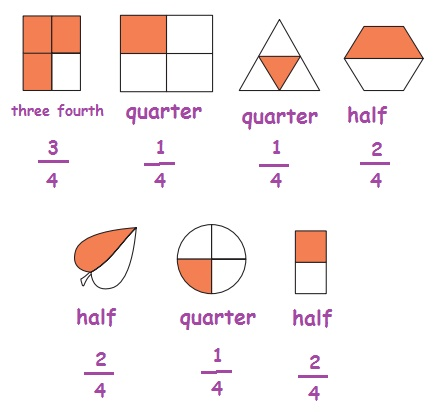Home | | Maths 4th Std | Exercise 6.4 (half, quarter, three fourth, semi, partial and whole)

# Exercise 6.4 (half, quarter, three fourth, semi, partial and whole)

Use the vocabulary as half, quarter, three fourth, semi, partial and whole

Exercise 6.4

I. Colour or shade half portion of the following pictures:II. Tick the picture that represent semi portion.III. Write appropriately which represent half, quarter, three fourth.Tags : Fraction | Term 2 Chapter 6 | 4th Maths , 4th Maths : Term 2 Unit 6 : Fraction
Study Material, Lecturing Notes, Assignment, Reference, Wiki description explanation, brief detail
4th Maths : Term 2 Unit 6 : Fraction : Exercise 6.4 (half, quarter, three fourth, semi, partial and whole) | Fraction | Term 2 Chapter 6 | 4th Maths